/

### Calculation of weighted moments and cumulants of probability distributions and samples

Equally-weighted statistics

The aggregate characteristics of probability distributions and data samples are commonly analysed using a small number of statistics corresponding to their first few moments, namely:

(a)    The mean of the distribution/sample,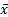, see MnMean, where: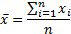(b)   The ‘sample’ and the ‘population’ variance,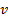and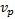respectively and the corresponding ‘sample’ and ‘population’ standard deviation,and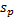of the distribution/sample, see MnVariance, MnPopulationVariance, MnStdev and MnPopulationStdev, where: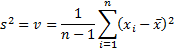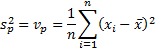In mathematical texts,is often referred to as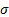andas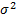.

(c)    The skew (i.e. ‘skewness’) of the distribution/sample,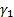, see MnSkew, where: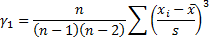(d)   The kurtosis  (or more precisely the ‘excess’ kurtosis),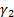, of the distribution/sample, see MnKurt, where: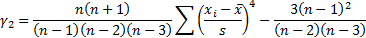We see that the ‘sample’ variance differs from the ‘population’ variance by a factor of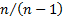representing the loss of one degree of freedom when calculating the mean. This adjustment is needed to ensure that the sample variance is an unbiased estimate of the underlying population variance if the distribution is Normal, for finite sized samples. In the large sample limit, i.e. where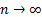, the two become equal.

The formula for the skew and kurtosis given above are properly ‘sample’ rather than ‘population’ measures. Both are dimensionless quantities, and thus invariant to changes in the ‘scale’ of the distribution (and its ‘location’) (i.e. if every element of the sample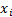was replaced by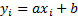, where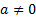(the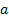representing a change of scale, and the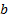representing a change in location) then the skew and kurtosis would remain unaltered. We could if we wished also define ‘population’ equivalents, see MnPopulationSkew, and MnPopulationKurt, where: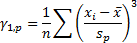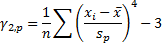Not equally-weighted statistics

In some circumstances different elements of a sample should be given different weights in the formulation of views regarding the overall probability distribution. For example, there may be greater errors known to be associated with some specific values used in constructing the sample, so less credibility should be attached to them when deciding on the overall shape of the distribution.

Given different weights,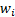to attach to each data point (which might, for example, be associated with the square of the standard error being ascribed to the relevant data point, say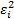), derivation of corresponding weighted ‘population’ statistics is relatively simple, e.g. the weighted mean,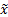, the weighted population variance,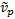, the weighted population standard deviation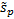, the weighted population skew,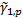, and the weighted population kurtosis,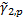, see MnWeightedMean, MnWeightedPopulationVariance, MnWeightedPopulationStdev, MnWeightedPopulationSkew and  MnWeightedPopulationKurt, may be defined as follows (dropping the explicit indexing of the summation element to simplify the formulae):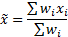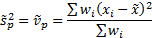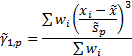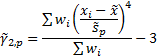More difficult is to identify the correct way to incorporate an appropriate small sample size adjustment to incorporate the right number of degrees of freedom. Different commentators (or at least software providers providing software downloadable from the Internet) appear to use different approaches, particularly when deriving a suitable measure of weighted ‘sample’ skew.

The Nematrian website adopts the approach that small sample size adjustment factors, at least  for the simpler moments/cumulants, should involve scale invariant factors that are reciprocals of expressions taking the following form (for suitable combinations of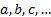where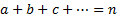,being the ‘order’ of the relevant measure) which reproduce the equally-weighted adjustment factors for cases where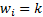for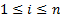and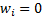for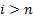: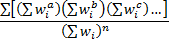This implies that the most appropriate definitions of the weighted sample variance,, the weighted sample standard deviationand the weighted sample skew,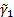, see MnWeightedVariance, MnWeightedStdev and MnWeightedSkew, are: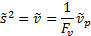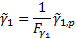where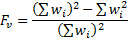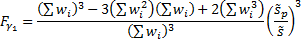Using this methodology it is less clear exactly what small sample size adjustment we should make when calculating a weighted (sample) kurtosis measure, but see Rimoldini (2013). In any case, some commentators such as Press et al. (2007) suggest that kurtosis (and skew) “should be used with caution, or better yet, not at all”. Kemp (2009) also questions the appropriateness of using skew and kurtosis to identify how non-Normal is a distributional form, see also TVaRForCubicQuantileQuantileRelationships. The corresponding Cornish-Fisher approximation that might otherwise be used to extrapolate the shape of the distributional form seems in general to give inappropriate weight to the wrong parts of the distributional form when assessing the extent of non-Normality.

Some software systems also allow users to calculate sample ‘moments’ relative to a predefined value, rather than the sample mean, but this is not currently possible using existing pre-defined Nematrian web service functions. It is not obvious to us whether it would be particularly useful in practice. For example, Press et al. (2007) note that using the formula defined above for the (equally-weighted) sample skew has a standard error, if the sample is drawn from a Normal distribution, of approximately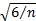. However, if we replace thein its definition by the true mean of the distribution then its standard error rises to approximately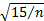. The corresponding approximate standard errors for kurtosis are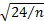and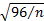respectively. Thus the computation of both skew and kurtosis becomes less accurate if we use the true mean in their formulae! For ease of reference, theandformulae are available directly using MnConfidenceLevelSkewApproxIfNormal and MnConfidenceLevelKurtApproxIfNormal.

Weighted correlation correficients, weighted covariances and weighted population covariances are defined in an equivalent manner, see MnWeightedCorrelations, MnWeightedCovariances and MnWeightedPopulationCovariances respectively.